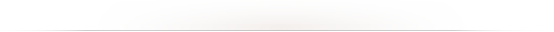signed

QiShunwang

“诚信为本、客户至上”

# 制作浮雕效果等高线的原理与实践

2020/12/26 21:58:52   来源：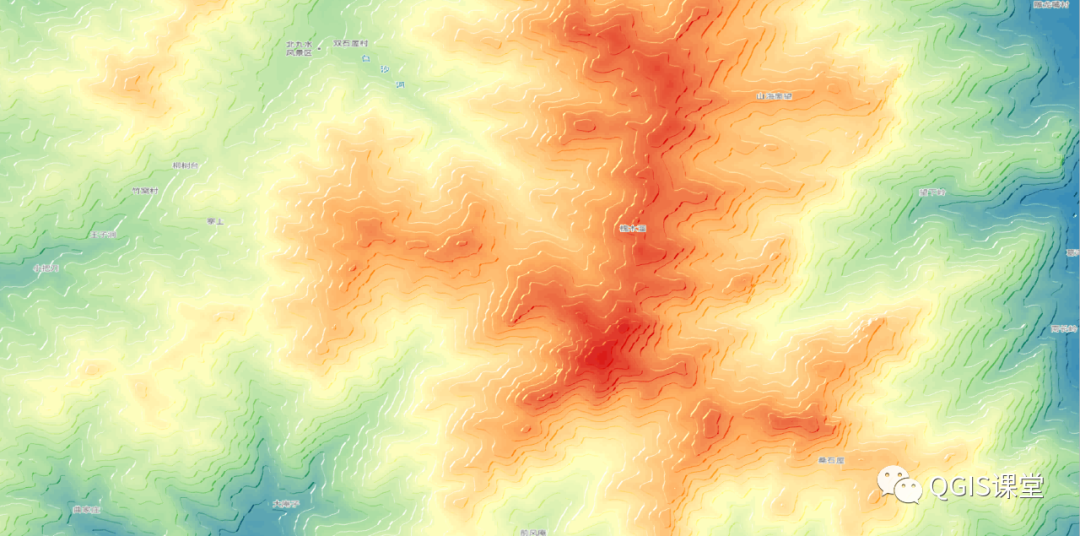# 01 准备数据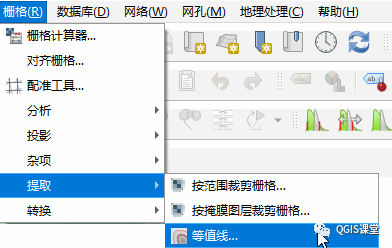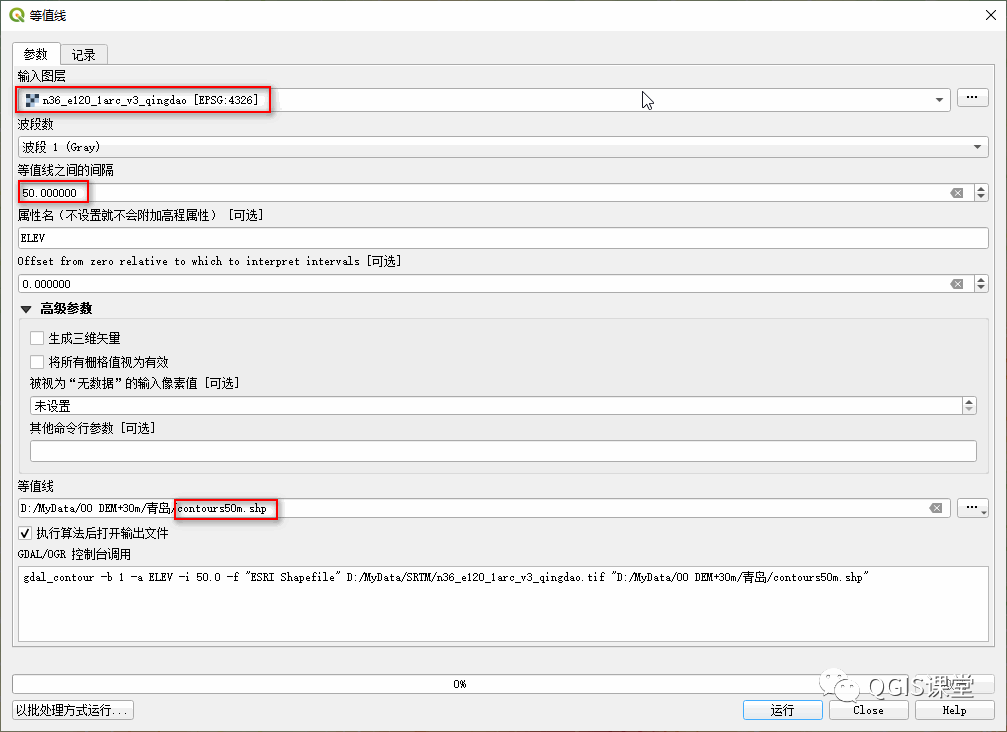# 02 下载并导入“Tanaka Contours”样式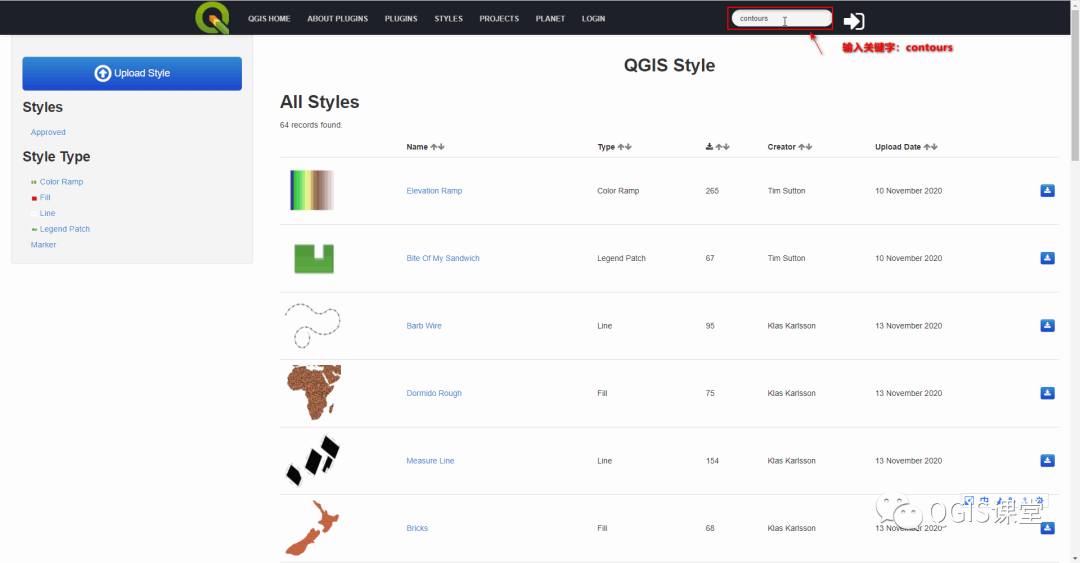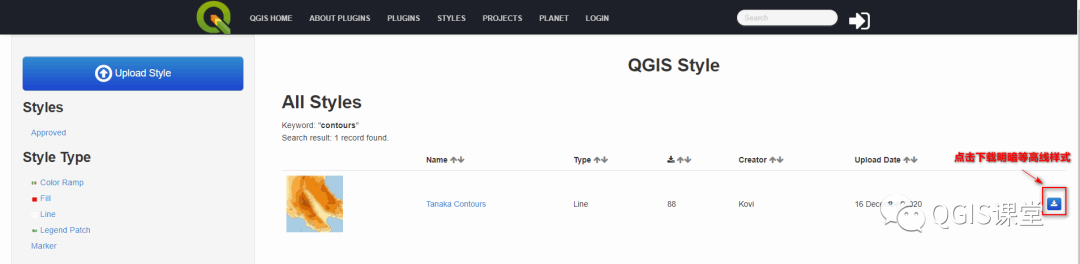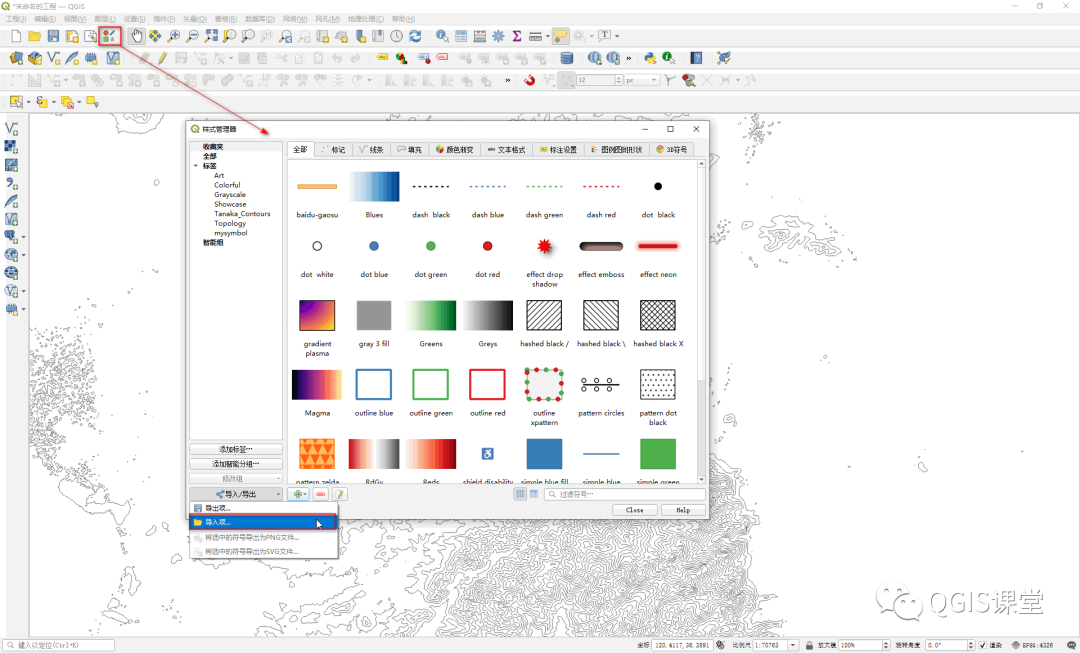# 03  配置明暗等高线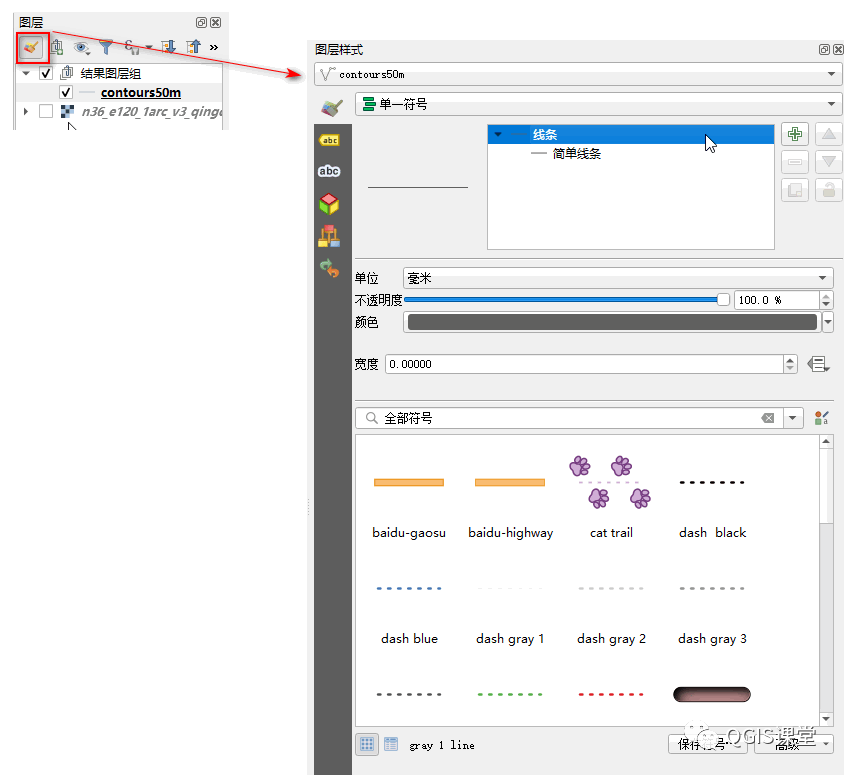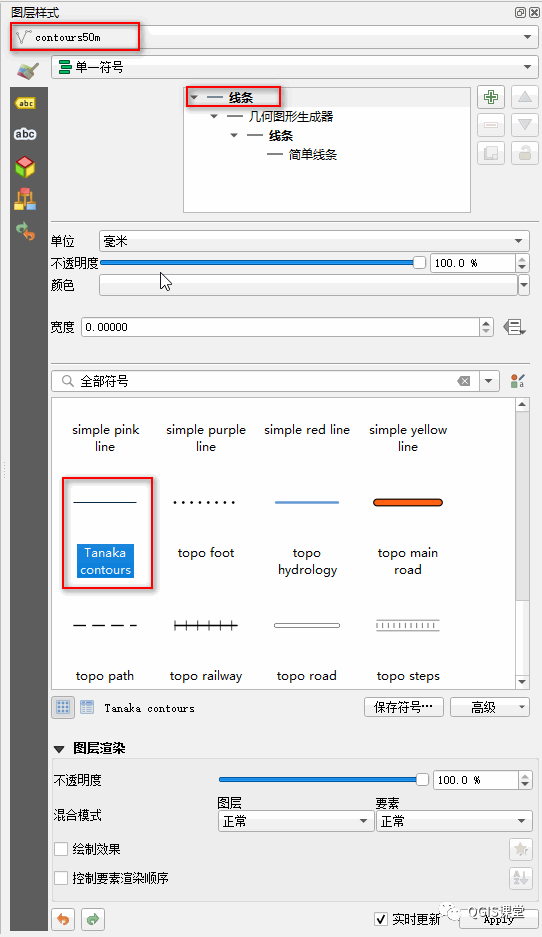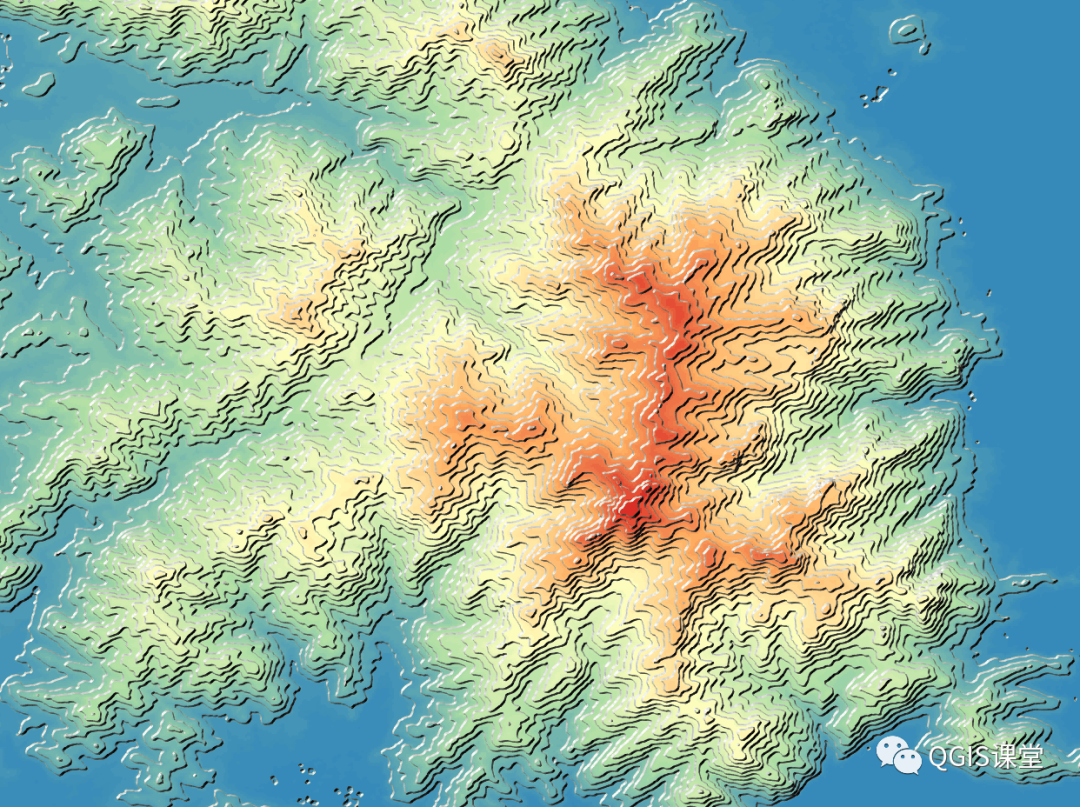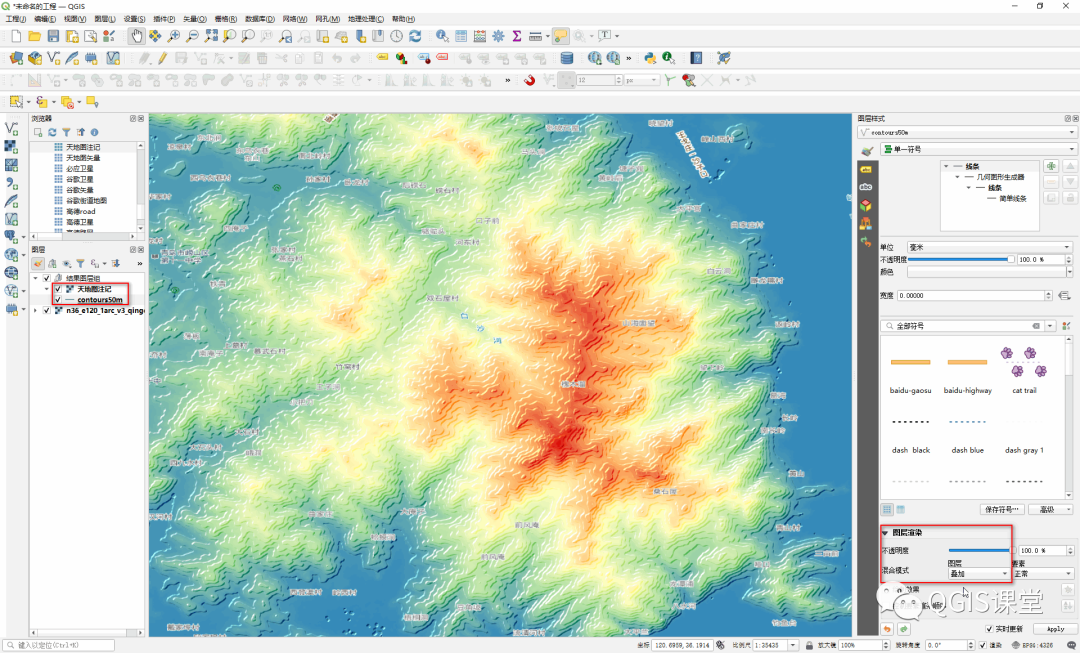# 04  实现原理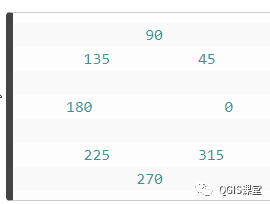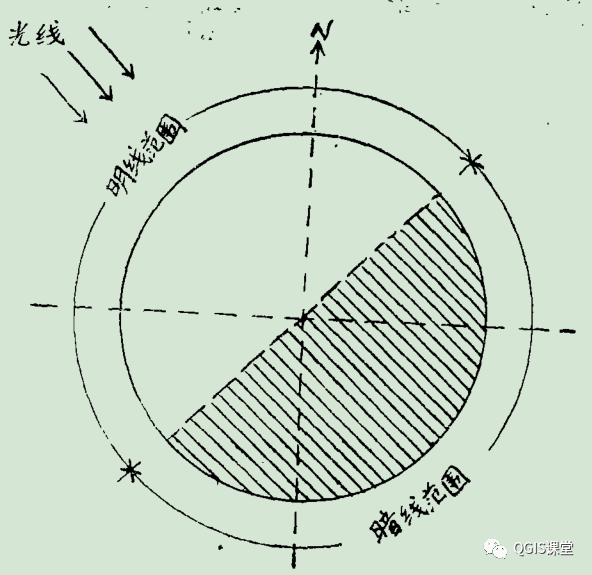• 第一步：用表达式将等高线提取为各个组成线段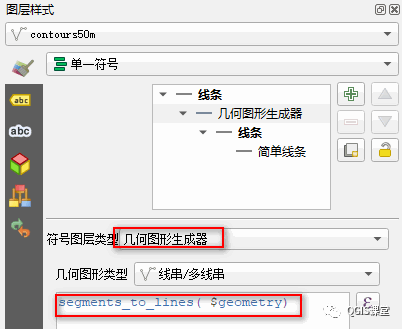“Tanaka contours”样式使用“几何图形生成器”将等高线的多个部分分解为单一线段，表达式为：

``segments_to_lines( \$geometry)``

• 第二步：根据线段的方位角计算出该段是处于明线范围还是暗线范围，并将角度映射为色调和线宽，刻画出立体效果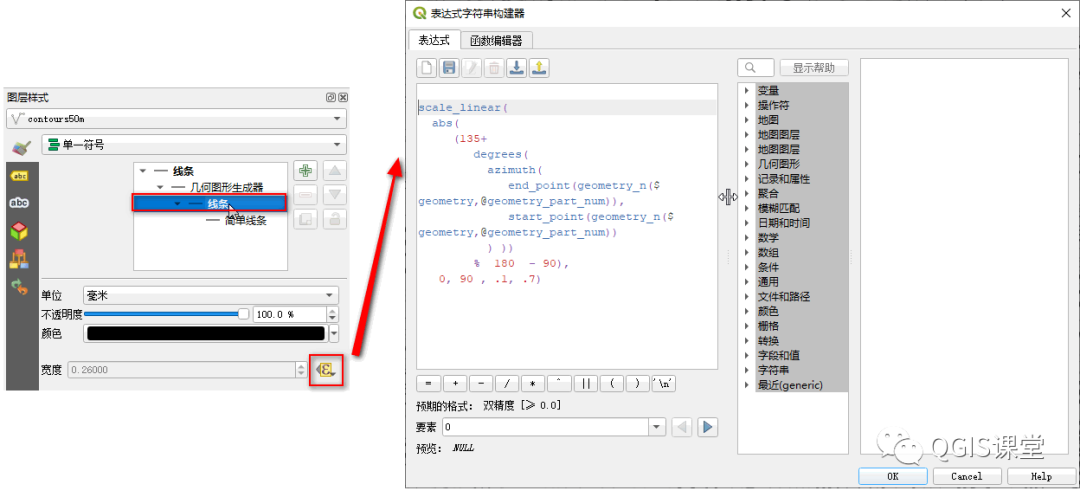``scale_linear(``  abs(``     (135+``        degrees(``          azimuth(``             end_point(geometry_n(\$geometry,@geometry_part_num)),``             start_point(geometry_n(\$geometry,@geometry_part_num))``      ) ))  ``        %  90),``   0, 90 , .1, .7)``

scale_linear：利用线性插值法将定义域中给定的值变换到某个输出范围，在本例中，scale_linear将[0,90]度角度映射成[0.1,0.7]线宽。

abs：取角度的绝对值。

degrees：将弧度转换为度。

azimuth：从垂直到终点（点A）到 起点（点B）上自北起顺时针方向量算的方位角的角弧度。

end_point：几何图形的最后一个结点。

start_point：几何图形的第一个结点。

geometry_n：从几何图形集合返回特定的几何图形；如果输入几何图形不是集合，则返回NULL。

\$geometry：当前要素的几何图形。

@geometry_part_num：变量，正在呈现的要素的当前几何图形部件编号。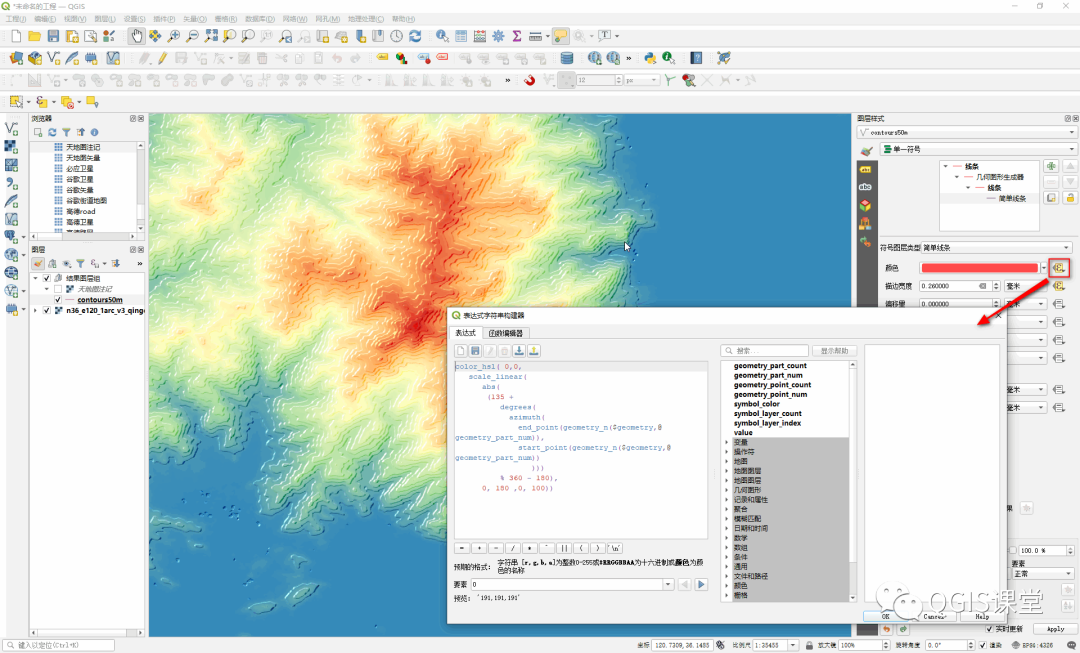``color_hsl( 0,0,``   scale_linear(``      abs(``       (135 +``          degrees(``            azimuth(``              end_point(geometry_n(\$geometry,@geometry_part_num)),``              start_point(geometry_n(\$geometry,@geometry_part_num))``                 )))  ``          % 360 - 180),``      0, 180 ,0, 100))``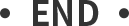https://anitagraser.com/2015/05/24/how-to-create-illuminated-contours-tanaka-style/。

 https://landscapearchaeology.org/2018/tanaka-contour-lines/。